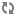Icehouse -  Icehouse -  Icehouse -  Icehouse -  Icehouse -  Icehouse -  Icehouse -  Icehouse -

### Database

Use the following options to alter Database-related settings.

Table 7.45. Description of configuration options for db
Configuration option = Default value Description
[DEFAULT]
sqlite_db = (StrOpt) The file name to use with SQLite
sqlite_synchronous = True (BoolOpt) If True, SQLite uses synchronous mode
[database]
backend = sqlalchemy (StrOpt) The backend to use for db
connection = sqlite:// (StrOpt) The SQLAlchemy connection string used to connect to the database
connection_debug = 0 (IntOpt) Verbosity of SQL debugging information. 0=None, 100=Everything
connection_trace = False (BoolOpt) Add python stack traces to SQL as comment strings
db_inc_retry_interval = True (BoolOpt) Whether to increase interval between db connection retries, up to db_max_retry_interval
db_max_retries = 20 (IntOpt) maximum db connection retries before error is raised. (setting -1 implies an infinite retry count)
db_max_retry_interval = 10 (IntOpt) max seconds between db connection retries, if db_inc_retry_interval is enabled
db_retry_interval = 1 (IntOpt) seconds between db connection retries
idle_timeout = 3600 (IntOpt) Timeout before idle sql connections are reaped
max_overflow = 20 (IntOpt) If set, use this value for max_overflow with sqlalchemy
max_pool_size = 10 (IntOpt) Maximum number of SQL connections to keep open in a pool
max_retries = 10 (IntOpt) Maximum db connection retries during startup. (setting -1 implies an infinite retry count)
min_pool_size = 1 (IntOpt) Minimum number of SQL connections to keep open in a pool
pool_timeout = 10 (IntOpt) If set, use this value for pool_timeout with sqlalchemy
retry_interval = 10 (IntOpt) Interval between retries of opening a sql connection
slave_connection = (StrOpt) The SQLAlchemy connection string used to connect to the slave database
use_db_reconnect = False (BoolOpt) Enable the experimental use of database reconnect on connection lost